#2017 iT 邦幫忙鐵人賽
DAY 28
5
Big Data

## Day28 R語言機器學習之K-NN、SVM、貝氏分類

• k最近鄰居分類法(k-Nearest Neighbour Classification)
• 支持向量機(Support Vector Machines, SVM)
• 樸素貝氏分類器(Naive Bayes Classifier)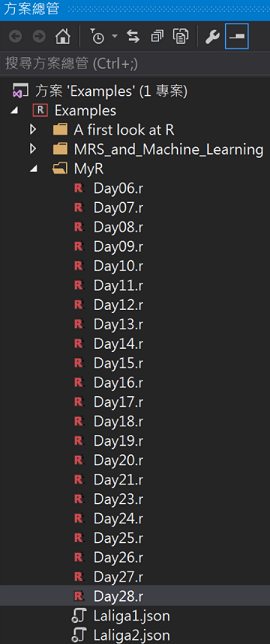``````#(1)載入iris資料
data(iris)

#(2)畫散佈圖
library(ggplot2)
#花萼長寬分佈
ggplot(iris, aes(x = Sepal.Length, y = Sepal.Width)) + geom_point(aes(color = Species))
#花瓣長寬分佈
ggplot(iris, aes(x = Petal.Length, y = Petal.Width)) + geom_point(aes(color = Species))
#(3)切分訓練及測試樣本
#設定亂數種子
set.seed(1111)
#樣本筆數
n <- nrow(iris)
#取出樣本數的idx
t_idx <- sample(seq_len(n), size = round(0.7 * n))
#訓練資料與測試資料比例: 70%建模，30%驗證
traindata <- iris[t_idx,]
testdata <- iris[ - t_idx,]
``````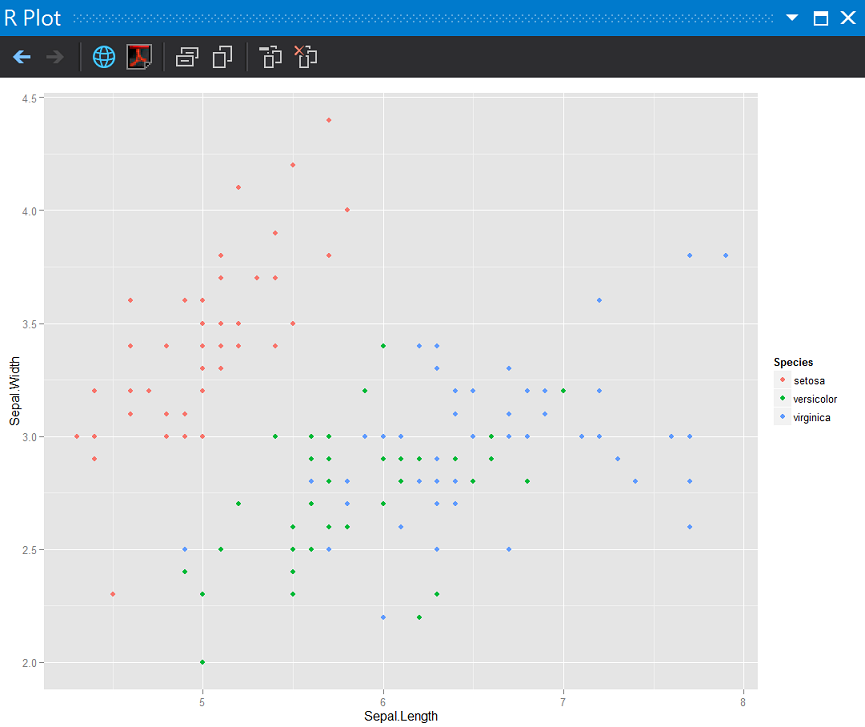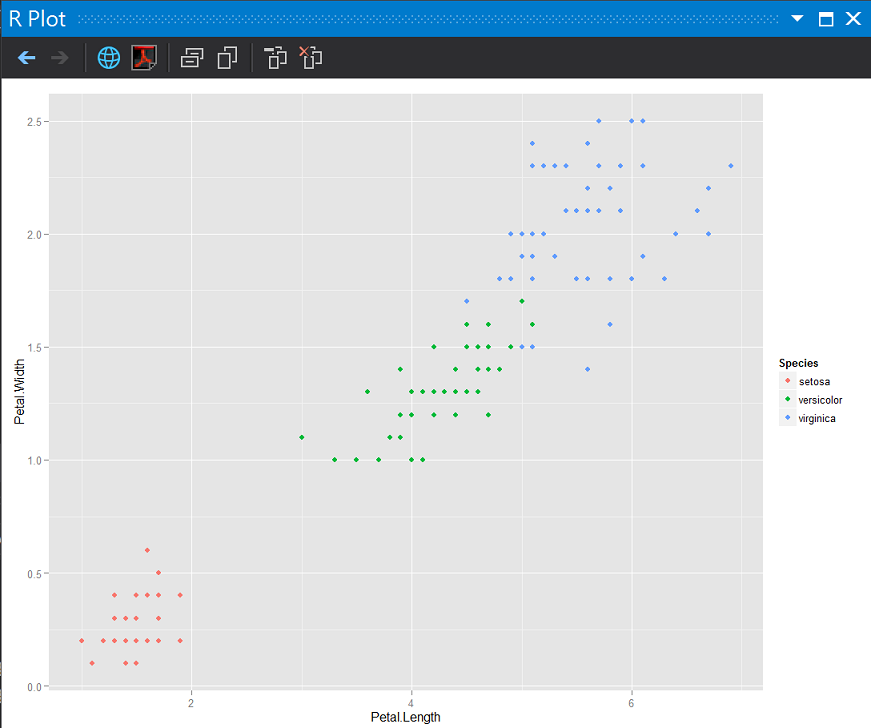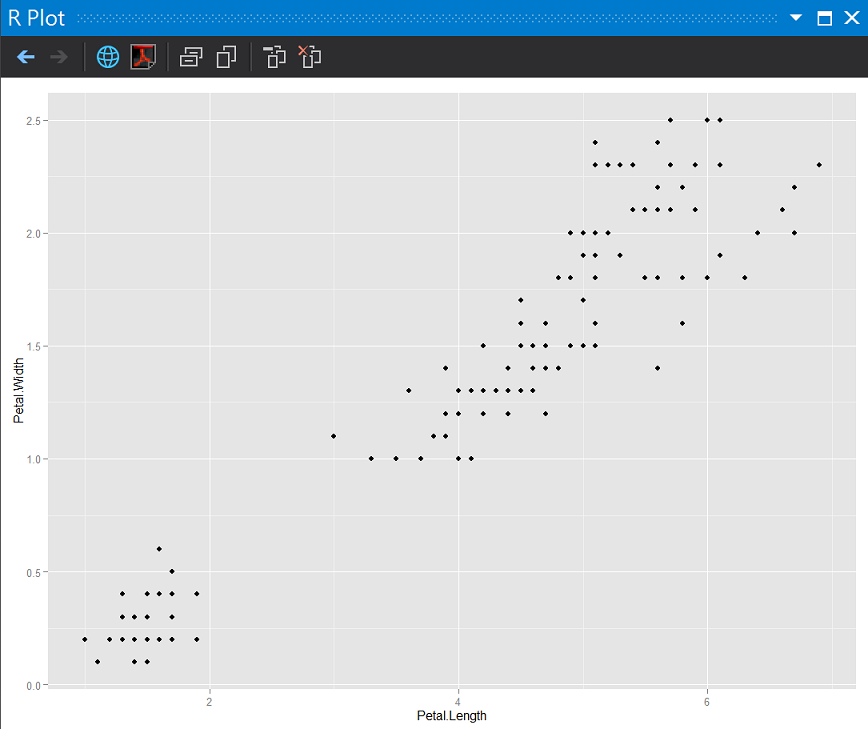## k最近鄰居分類法(k-Nearest Neighbour Classification)

K-NN使用點間的距離為分類標準，當新的觀測值準備預測時，演算法會計算出和她接近最多的類目標點，比方說，k值=5，觀測值比較靠近其中3個目標點時，演算法就會採用多數票來決定分類結果。

``````#安裝並載入class套件
library(class)
library(dplyr)
#(參數1)準備訓練樣本組答案
trainLabels <- traindata\$Species

#(參數2)(參數3)去除兩個樣本組答案
knnTrain <- traindata[, - c(5)]
knnTest <- testdata[, - c(5)]

#計算k值(幾個鄰居)通常可以用資料數的平方根
kv <- round(sqrt(n))
kv

#(4)建立模型
prediction <- knn(train = knnTrain, test = knnTest, cl = trainLabels, k = kv)

#(5)評估正確性
cm <- table(x = testdata\$Species, y = prediction, dnn = c("實際", "預測"))
cm

knnaccuracy <- sum(diag(cm)) / sum(cm)
knnaccuracy
``````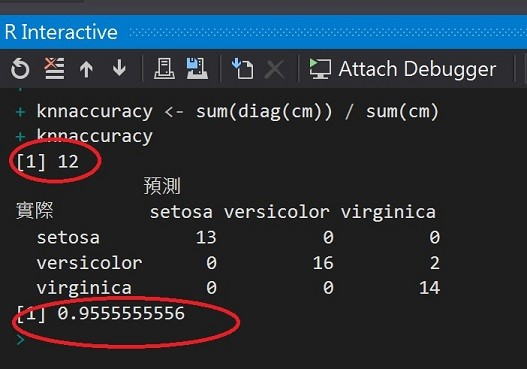``````#利用knn結果畫圖
#訓練組
knnTrain\$Species <- trainLabels
ggplot(knnTrain, aes(x = Petal.Length, y = Petal.Width)) + geom_point(aes(color = Species)) +
stat_density2d(aes(color = Species))

#測試組
knnTest\$Species <- prediction
ggplot(knnTest, aes(x = Petal.Length, y = Petal.Width), n = 10) + geom_point(aes(color = Species)) +
stat_density2d(aes(color = Species), h = 0.6)
``````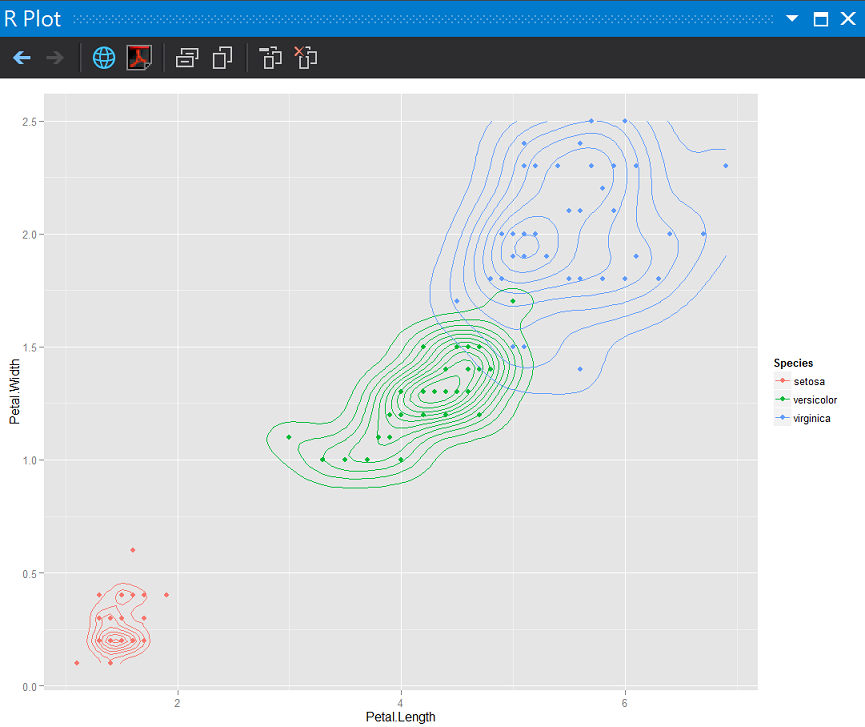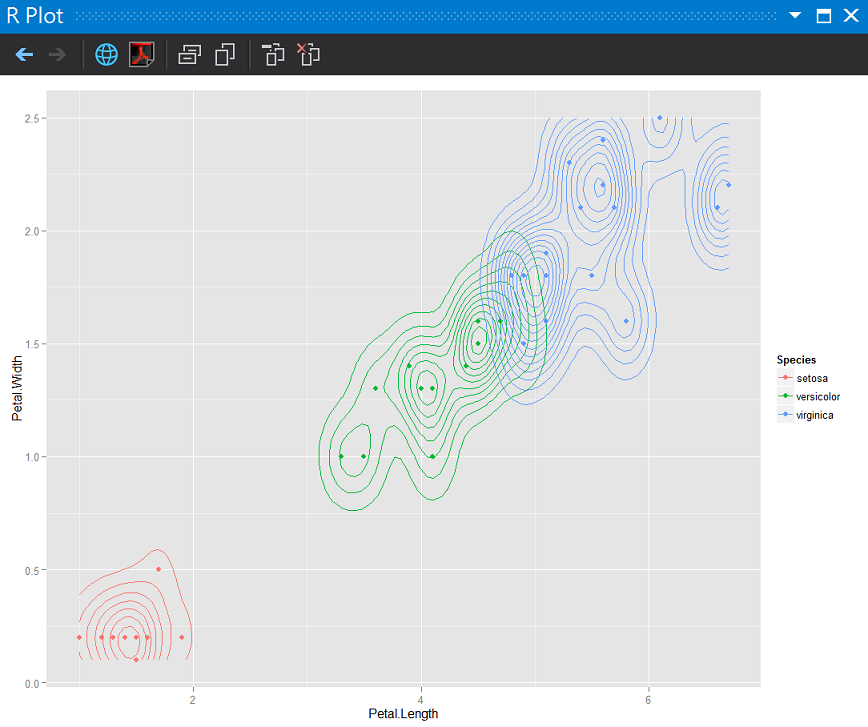Knn這次預測的問題在virginica和versicolor兩個亞種同時位在DMZ時(分佈太接近)，會有誤判。

## 選擇k value

k值太小會有overfitting的問題，思考了一下1-nn的概念，如果每個訓練用的觀測值都自成一格，在分類上的效果好像就失去了，所以也許比較的範圍可以從2-2k

``````# 選擇k value
klist <- seq(1:(kv + kv))
knnFunction <- function(x, knnTrain, knnTest, trainLabels, testLabels) {
prediction <- knn(train = knnTrain, test = knnTest, cl = trainLabels, k = x)
cm <- table(x = testLabels, y = prediction)
accuracy <- sum(diag(cm)) / sum(cm)
}
accuracies <- sapply(klist, knnFunction, knnTrain = knnTrain, knnTest = knnTest, trainLabels = trainLabels, testLabels = testdata\$Species)

# k value與準確度視覺化
df <- data.frame(
kv = klist, accuracy = accuracies)

ggplot(df, aes(x = kv, y = accuracy, label = kv, color = accuracy)) +
geom_point(size = 5) + geom_text(vjust = 2)

``````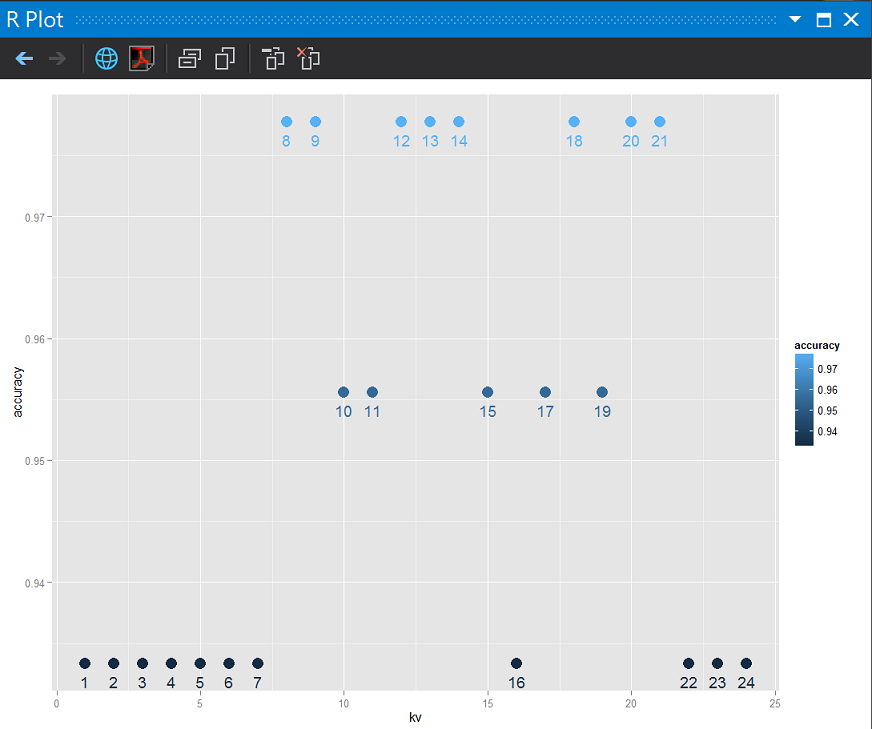## 支持向量機(Support Vector Machines, SVM)

SVM WIKI

``````#載入套件
library(e1071)

# 建立模型
svmM <- svm(Species ~ ., data = traindata, probability = TRUE)

# 預測
results <- predict(svmM, testdata, probability = TRUE)

# 評估
cm <- table(x = testdata\$Species, y = results)
cm
SVMaccuracy <- sum(diag(cm)) / sum(cm)
SVMaccuracy
``````## 樸素貝氏分類器(Naive Bayes Classifier)

``````nbcm <- naiveBayes(Species ~ ., data = traindata)
results <- predict(nbcm, testdata)

# 評估
cm <- table(x = testdata\$Species, y = results)
cm
naiveBayesaccuracy <- sum(diag(cm)) / sum(cm)
naiveBayesaccuracy
``````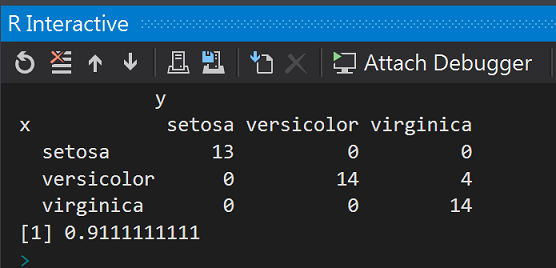## 漢堡王之今日我最大

``````df <- data.frame(perf = c(knnaccuracy, SVMaccuracy, naiveBayesaccuracy), name = c("KNN", "SVM", "naiveBay"));

ggplot(df, aes(x = name, y = perf, color = name, label = perf)) +
geom_point(size = 5) + geom_text(vjust = 2)
``````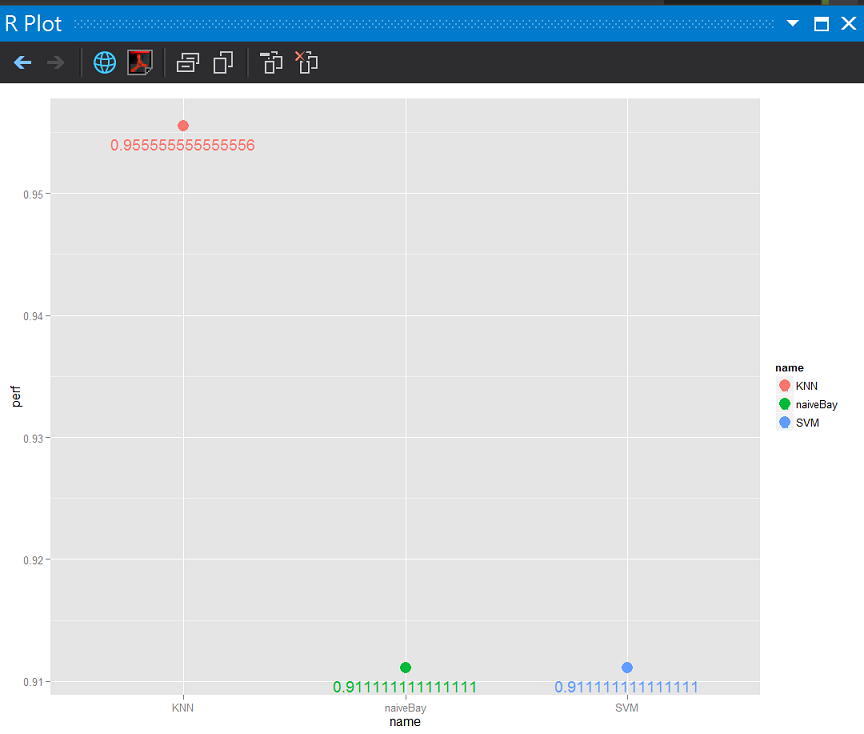## 參考:2013.05攝於Český Krumlov,Czech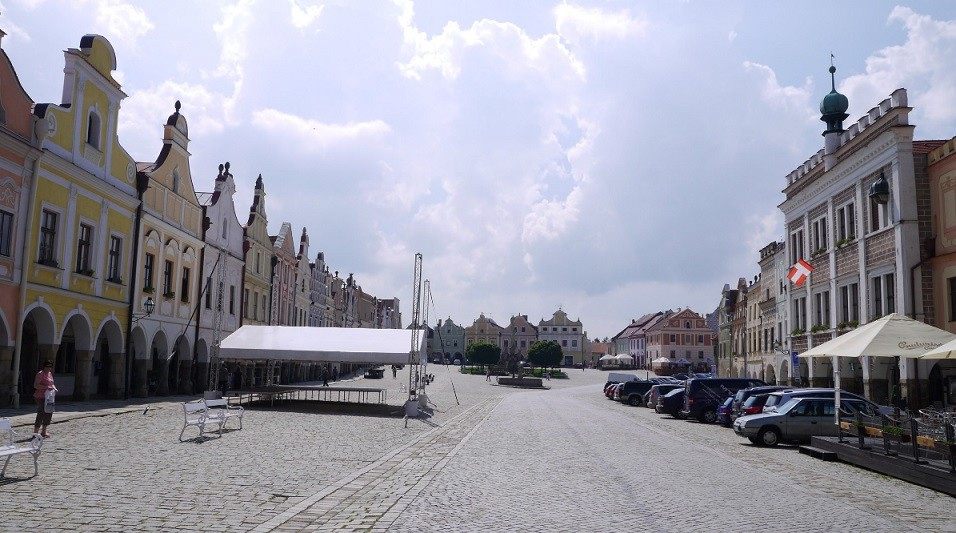2013.05攝於telc,Czech

R語言與機器學習見面會30